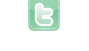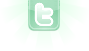## Pulling items out of lists troubles

I am having problems with comparing elements of an Integer ArrayList (single dimension) with certain values. Is there anyone who sees what I'm doing wrong? Here I declare the new lists:
``` private List listx1 = new ArrayList<Integer>();
private List listy1 = new ArrayList<Integer>();
private List listx2 = new ArrayList<Integer>();
private List listy2 = new ArrayList<Integer>();```
Here I add numbers to them:
```        else if(Greenfoot.isKeyDown("enter") && canPlace() && !menuTime)
{
int e = getX();
int f = getY();
if(player==1)
{
setImage("naught-small.png");
if(doesWin(listx1) || doesWin(listy1) || diagonalWin(listx1, listy1))
System.out.print("Hooray! Crosses wins!\n");
wait(20);
player = 2;
}
else
{
setImage("cross-small.png");
if(doesWin(listx2) || doesWin(listy2) || diagonalWin(listx2, listy2))
System.out.print("Hooray! Naughts wins!\n");
wait(20);
player = 1;
}
}```
Here I check (very inefficiently probably) if there are 3 of the same items in the list:
```    public boolean doesWin(List x)
{
Collections.sort(x);
if(Collections.frequency(x, 100) == 3 || Collections.frequency(x, 300) ==3 || Collections.frequency(x, 500) == 3)
return true;
else
return false;
}```
And here I check for a diagonal win, so that would mean I have a same x and y, with 3 different values. In the contains method it goes wrong at the line "int placeholder = x.get(i);" I get the error "incompatible types: jave.lang.Object cannot be converted to in".
```    public boolean diagonalWin(List x, List y)
{
if(contains(x, 100) && contains(y,100))
return true;
return false;
}

public boolean contains(List x, int z)
{
for(int i=0; i<x.size(); i++)
{
int placeholder = x.get(i);
}
return false;
}```
Every bit of help is welcome, as I searched the web for over an hour for a solution already but I can't find it.
Very inefficient. Use a 3 by 3 int array to represent the grid, 0 representing an empty square, and 1 and -1 representing the crosses and naughts. The 1s and -1s can come from a turn field representing whose turn it is. It would be quite easy to check for a win with the array set up this way.
Yes, but how do you take those items out of the list to compare them?
Windeycastle wrote...
Yes, but how do you take those items out of the list to compare them?
```// global declarations
int[][] grid = new grid; // grid array
int turn = 1; // player at turn (1 for crosses; -1 for naughts)

// in act of world
if (numberOfObjects() < 9 && Greenfoot.mouseClicked(this))
{
MouseInfo mi = Greenfoot.getMouseInfo();
int x = mi.getX(), y = mi.getY();
if (grid[y][x] != 0) return; // do nothing if grid square occupied
grid[y][x] = turn; // make entry
addObject((turn == 1 ? new Cross() : new Naught()), 100+200*x, 100+200*y);
if (doesWin(x, y)) gameOver(turn);
else if (numberOfObjects() == 9) gameOver(0);
turn = -turn;
}

// to tell is current turn wins
private boolean doesWin(int x, int y)
{
int n = grid[y][x];
if (n == grid[y][(x+1)%3] && n == grid[y][(x+2)%3]) return true; // row check
if (n == grid[(y+1)%3][x] && n == grid[(y+2)%3][x]) return true; // column check
if (x == y && n == grid[(y+1)%3][(x+1)%3] && n == grid[(y+2)%3][(x+2)%3]) return true; // back-slash diagonal check
if (x+y == 2 && n == grid[(y+1)%3][(x+2)%3] && n == grid[(y+2)%3][(x+1)%3]) return true // forward-slash diagonal check
return false; // no win found
}

// game over routine
private void gameOver(int winner)
{
String txt = null;
switch (winner)
{
case 1 : txt = "Crosses\nwins"; break;
case -1 : txt = "Naughts\nwins"; break;
case 0 : txt = "Draw\ngame"; break;
}
GreenfootImage img = new GreenfootImage(txt, 60, new Color(0, 0, 0, 128), new Color(0, 0, 0, 0));
getBackground().drawImage(img, 300-img.getWidth()/2, 300-img.getHeight()/2);
}```
This is the answer I've been looking for!! Thank you sl much, I will use all of your ideas here but ttry to implement them a bit different so it is still something I made and not your copy, but I'll set you definitly in the documentation =)
I found an inconsistency in my code. If world size is (600, 600, 1), line 9 should have x and y divided by 200. If world size is (3, 3, 200), line 12 should use just x and y.
I did a complete rarrangement and changed the rediculous world size of 600,600,1 to 1,1,200. Makes a lot of code easier and more understandable.
Windeycastle wrote...
I did a complete rarrangement and changed the rediculous world size of 600,600,1 to 1,1,200. Makes a lot of code easier and more understandable.
You do mean (3, 3, 200), don't you?
Yes (insert facepalm)
Hmm, working on the program now, but it gives an error when declaring the array? It says "unknown type: grid" at the right side of the "=".
I think it should be
`int[][] grid = new int;`